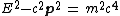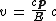# tachyon

(redirected from Imaginary mass)
Also found in: Dictionary.

## tachyon

(tăk`ēŏn'), hypothetical elementary particleelementary particles,
the most basic physical constituents of the universe. Basic Constituents of Matter

Molecules are built up from the atom, which is the basic unit of any chemical element. The atom in turn is made from the proton, neutron, and electron.
that travels only at speeds exceeding that of light. According to the theory of relativityrelativity,
physical theory, introduced by Albert Einstein, that discards the concept of absolute motion and instead treats only relative motion between two systems or frames of reference.
, the speed of light is the limiting velocity for all ordinary material particles. Particles having nonzero rest mass can approach, but not reach, the speed of light, since their mass would become infinite at that speed. On the other hand, particles with zero rest mass, such as the photonphoton
, the particle composing light and other forms of electromagnetic radiation, sometimes called light quantum. The photon has no charge and no mass. About the beginning of the 20th cent.
, must always travel at the speed of light; they cannot be brought to rest or even slowed down. Theorists have argued that since nothing in principle prohibits the existence of a third class of particles that travel only at speeds exceeding that of light, such particles, called tachyons [Gr. tachys,=swift] may exist although no evidence for them has been found. In the terminology of the theory, the particles that travel only at the speed of light are called luxons, and those that travel at lesser speeds are called tardyons. Like the original theory of relativity, the theory of tachyons has several aspects that appear to contradict common sense but that are fully self-consistent. For example, a tachyon must have an imaginary (in the mathematical sense) rest mass, or proper mass, and it must travel faster rather than slow down when it loses energy.

## Tachyon

A hypothetical faster-than-light particle consistent with the special theory of relativity. According to this theory, a free particle has an energy E and a momentum p which form a Lorentz four-vector. The length of this vector is a scalar, having the same value in all inertial reference frames. One writes

(1)Eq. (1), where c is the speed of light and the parameter m2 is a property of the particle, independent of its momentum and energy. Three cases may be considered: m2 may be positive, zero, or negative. The case m2 > 0 applies for atoms, nuclei, and the macroscopic objects of everyday experience. The positive root m is called the rest-mass. If m2 = 0, the particle is called massless. A few of these are known: the electron neutrino, the muon neutrino, the photon, and the graviton. The name tachyons (after a Greek word for swift) has been given to particles with m2 < 0.

In general, the particle speed is given by Eq. (2). If m2 < 0,

(2)Eq. (1) implies E > c p and Eq. (2) gives v < c. If m2 = 0, then E = c p and v = c. In case m2 < 0, one finds E < c p and v > c. Tachyons exist only at faster-than-light speeds. See Elementary particle, Relativity

McGraw-Hill Concise Encyclopedia of Physics. © 2002 by The McGraw-Hill Companies, Inc.

## tachyon

[′tak·ē‚än]
(particle physics)
A hypothetical particle that travels faster than light, consistent with the theory of relativity.
McGraw-Hill Dictionary of Scientific & Technical Terms, 6E, Copyright © 2003 by The McGraw-Hill Companies, Inc.
Mentioned in ?
References in periodicals archive ?
(4) Using the Feynman path integral (PI) approach to quantum field theory that includes particles emerging from the vacuum going faster-than-light, I speculate that there are two basic quantum fields: a real Higgs field capable of generating real positive mass particles (fermions) and an imaginary Higgs field capable of generating mind-like imaginary mass particles (tachyons).
K is in general a complex number thus giving rise to an imaginary mass component for the last two terms.
Because tachyons have imaginary masses, they are confined to speeds in excess of light; they may be seen to play a role in memory processes and a role in the feeling of intent following the orderly and massive process of thinking.
An imaginary mass transfer of 5% of the total mass was carried out from acetone extract [i.e., (5/7) x 100 = 0.71 of acetone extract] to the rubber fraction of TGA.
Here is the answer: [r.sub.g] is the photometric distance in the radial direction, separating the "external" region inhabited with real particles and the "internal" region under the radius wherein all particles bear imaginary masses. Particles which inhabit the boundary surface (its radius is [r.sub.g]) bear singular physical properties.
4.2 Hypothetical imaginary mass problem in Klein-Gordon equation
Nonetheless, in this section we can reveal that perhaps the problem of imaginary mass in Klein-Gordon equation is not completely avoidable.
Therefore we can conclude that imaginary mass term appears in the definition of coefficient [k'.sub.0] of this new Klein-Gordon equation.
This extended physics grants the mass a complex nature implying the possible existence of purely real masses [+ or -]m and purely imaginary masses: [+ or -] [(-1).sup.1/2] m.
They do not, partly because of their fear of alienating those future imaginary masses that will supposedly line up behind them, and partly because this front includes many ex- and non-MB activists who will never advocate or accept those "radical" positions as their own.

Site: Follow: Share:
Open / Close